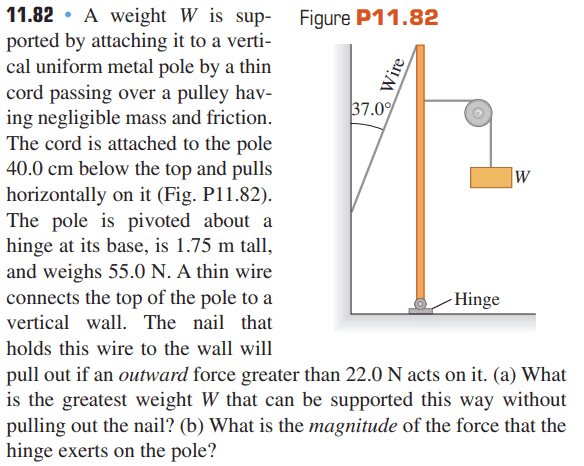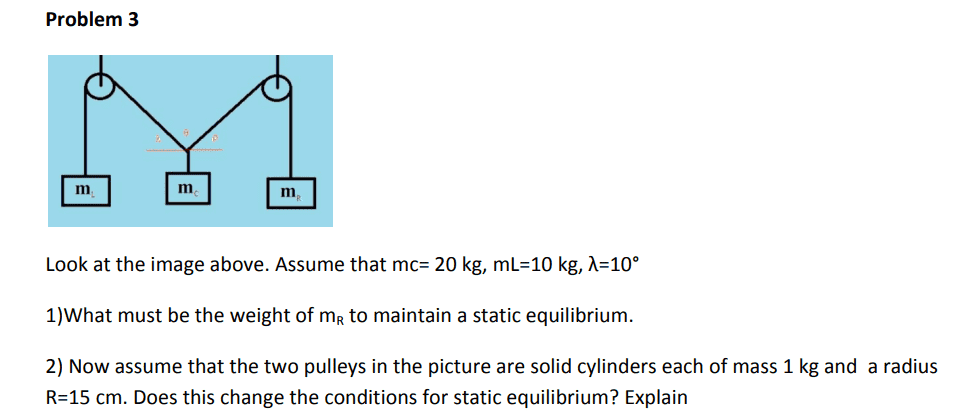# Help with understanding this rigid-body equilibrium problem

Kolika28
Homework Statement:
I have a hard time understanding rigid-body equilibrium problem, especially when it a system of several objects. Sorry for asking so much here, but my exam is coming up and I really want to make sure that I try to understand all the things that makes me confussed.
Relevant Equations:
##\sum F_x=0##
##\sum F_y=0##
##\sum \tau=0##
So here are two problems I have been working with lately:I have solved both, so I don't need the help to find the answers. The thing that confusses me is which object one should choose to apply the equations above. When it comes to the first problem, we apply the equations to the pole, but why not the box? And in second problem, we apply them to the point where the three strings meet. I had to look at the solution to find out which object or point I should focus on. But I'm afraid it won't be obvious to me on the exam what object or point to pick. Does anyone have some tips?

Homework Helper
which object one should choose
...
some tips?
Make free body diagrams. The ones for W, pulley or wire in the first problem and the ones for the masses and the pulleys in the second problem will be boring. The obvious key candidates will pop up pretty soon.

•Kolika28
rjurga
For torques:

You can compute torques about any point you wish. Given that freedom, you should pick the point that will lead to the easiest computations. In particular, if a force is acting at a point, and you compute torques about that same point, that force will not contribute a torque because the distance to the point is zero, so that's one torque less to worry about. Such simplifications are the first things I look for when choosing where I'm computing torques about.

For forces:

In the first problem, I would not bother writing it down for the box, because it's in a simple enough situation where you can figure out the tension in the string in your mind.

Same for box 1 and 3 in the second problem.

In an exam, you might still want to write it down just to show the person who will correct that you understood.

•Kolika28, Lnewqban and Delta2
Homework Helper
Gold Member
Homework Statement:: I have a hard time understanding rigid-body equilibrium problem, especially when it a system of several objects. Sorry for asking so much here, but my exam is coming up and I really want to make sure that I try to understand all the things that makes me confused.
...
The thing that confusses me is which object one should choose to apply the equations above. When it comes to the first problem, we apply the equations to the pole, but why not the box? And in second problem, we apply them to the point where the three strings meet. I had to look at the solution to find out which object or point I should focus on. But I'm afraid it won't be obvious to me on the exam what object or point to pick. Does anyone have some tips?

These problems require practice, time and patience.

Normally, the questions will guide you to the object which forces you need to analyze, decompose in their x and y components and later calculate.
If you apply the equations to the wrong object or point of a mechanism, you soon will understand that such choice is not taking you too far.

Nothing lost at that point, other than time, you keep going with other points and keep collecting information that will help you determine the magnitudes and directions of forces and moments.

•Kolika28 and Delta2
Kolika28
Thank you so much everybody for your relplies, I really appreciate it!I will now try to solve some more problems like this with your comments in mind, and see if I understand it better now.

•PhDeezNutz